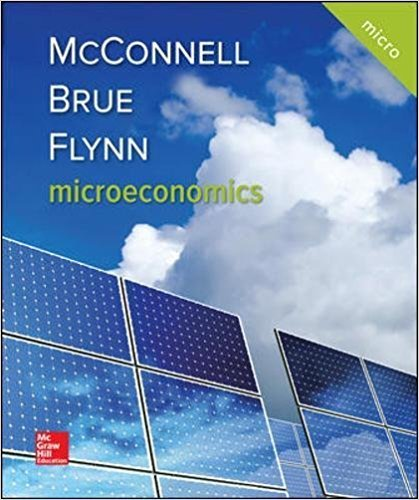×
×

# Solutions for Chapter 20: Public Finance: Expenditures and Taxes## Full solutions for Microeconomics | 21st Edition

ISBN: 9781259915727Solutions for Chapter 20: Public Finance: Expenditures and Taxes

Solutions for Chapter 20
4 5 0 407 Reviews
28
2
##### ISBN: 9781259915727

This expansive textbook survival guide covers the following chapters and their solutions. Chapter 20: Public Finance: Expenditures and Taxes includes 13 full step-by-step solutions. Microeconomics was written by and is associated to the ISBN: 9781259915727. Since 13 problems in chapter 20: Public Finance: Expenditures and Taxes have been answered, more than 6829 students have viewed full step-by-step solutions from this chapter. This textbook survival guide was created for the textbook: Microeconomics, edition: 21.

Key Business Terms and definitions covered in this textbook
• average total cost

total cost divided by the quantity of output

• bond

a certificate of indebtedness

fluctuations in economic activity, such as employment and production

• commodity money

money that takes the form of a commodity with intrinsic value

• cost

the value of everything a seller must give up to produce a good

• equilibrium quantity

the quantity supplied and the quantity demanded at the equilibrium price

• fractional-reserve banking

a banking system in which banks hold only a fraction of deposits as reserves

• inferior good

a good for which, other things being equal, an increase in income leads to a decrease in demand

• leverage

the use of borrowed money to supplement existing funds for purposes of investment

• liquidity

the ease with which an asset can be converted into the economy’s medium of exchange

• marginal rate of substitution

the rate at which a consumer is willing to trade one good for another

• market failure

a situation in which a market left on its own fails to allocate resources efficiently

• profit

total revenue minus total cost

• public goods

goods that are neither excludable nor rival in consumption

• public saving

the tax revenue that the government has left after paying for its spending

• quantity equation

the equation M × V = P × Y, which relates the quantity of money, the velocity of money, and the dollar value of the economy’s output of goods and services

• real GDP

the production of goods and services valued at constant prices

• total revenue (for a firm)

the amount a firm receives for the sale of its output

• transaction costs

the costs that parties incur in the process of agreeing to and following through on a bargain

• vertical equity

the idea that taxpayers with a greater ability to pay taxes should pay larger amounts

×